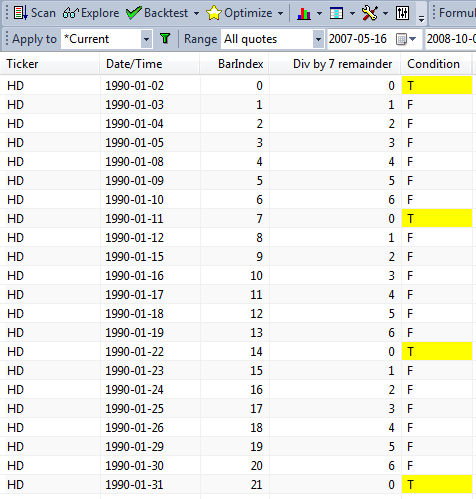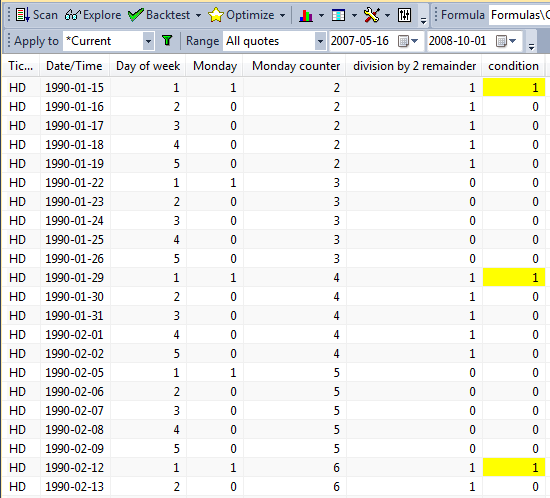amibroker

Detecting N-th occurrence of a condition using modulus operator

Modulus (%) is an operator that returns the reminder from integer division. It is very helpful to create counters that wrap-around at user-specified N.

In order to define a condition, which returns True every Nth bar, the easiest way is just to use % (modulus) operator. If we apply modulus to consecutive numbers such as BarIndex() – then calculating the reminder from integer division of barindex by N will return 0 every Nth bar (on bars that are divisible by N). We can use the following exploration to demonstrate that:

7;
bi BarIndex();
condition bi == 0;

Filter 1;
AddColumnbi "Div by 7 remainder ");

formatCharcolorDefaultIIfconditioncolorYellowcolorDefault ) )Since the remainder from division by 7 will equal zero only for the multiples of 7, then we will have our condition True every 7th bar (as marked in the above exploration results with T letter on yellow background).

Using the same technique we can also count occurrences of certain criteria and then apply the % operator. For example – let us say we want to test a rotational strategy, where we rotate our portfolio every 2nd Monday. To detect such condition in our code we need to first identify all Monday bars, then count them and use % operator to divide such count by 2.

The following exploration shows the calculations of the condition we look for:

Filter 1;
AddColumncountMon == 0"division by 2 remainder");Here is the formula showing how to code these technique for the rotational back-test:

SetBacktestModebacktestRotational );
posScore 100 RSI(); // sample scoring indicator
SetPositionSize10spsShares ); // sample position sizing

Mon DayOfWeek() == 1// identify Mondays
countMon CumMon ); // count Mondays

// rotate only on Monday, every 2nd one
rotation Mon AND (countMon == );
PositionScore IIfrotationposScorescoreNoRotate )